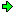WON plate71 | World!OfNumbers[ February 21, 2000 ] Arranging all the base b representations of palindrome 31113 Concatenating all the base b representations of palindrome 31113 in an upward (2<=b<=10) and downward (10>=b>=2) string produces two genuine primes. Prime 1 = 3111346610746111564654000131443423132120211120200100111100110001001 Prime 2 = 1111001100010011120200100132120211443423400013156465746114661031113 The following palindrome with this property is 73837. Both 31113 and 73837 are composite palindromes. So, who can provide me the first palindromic prime example ?Twenty two years later [ February, 2022 ] I wrote a Pari/gp program with the purpose to search for myself that first palindromic prime example. ```\\ Patrick De Geest [ GP/PARI CALCULATOR Version 2.11.4 ] February 7, 2022 { forstep(a=1,oo,2, t=digits(a); if(Vecrev(t)==t, spup=[]; spdo=[]; marque=0; for(i=2,10, spup=concat(spup,digits(a,i)); spdo=concat(digits(a,i),spdo) ); if(isprime(fromdigits(spup)) && isprime(fromdigits(spdo)), marque=1; print(fromdigits(spdo));print(fromdigits(spup));print()); if(isprime(a)&&marque, print(a," is a palindromic PRIME!"); break()); ); ); } ``` On [ February 10, 2022 ] after running the code for a few hours the following appeared on my screen 98802520889is a palindromic PRIME! Prime 1 = 98802520889313021332645134010555147110065263366522 \ 11322002453010531043214211320241130001011231030321 \ 1001100002011010022011121011100000001000101101101001100111001 Prime 2 = 1011100000001000101101101001100111001100110000201101002201112 \ 11300010112310303213104321421132024113220024530105 \ 10065263366522134010555147131302133264598802520889 Note that the primes formed by the concatenations is of palindromic length itself namely161 !Direct OEIS References A054258 Concatenation of n in base 2 up to base 10 and n in base 10 down to base 2 is prime,all numbers are interpreted as decimals. A054257 Concatenation of n in base 10 down up to base 2 is prime,all numbers are interpreted as decimals. A054256 Concatenation of n in base 2 up to base 10 is prime, all numbers are interpreted as decimals. Indirect OEIS References A054219 Concatenation of n in base 10 down to base 2 is divisible by at least one of these base b numbers,all numbers interpreted as decimals. A054220 Concatenation of n in base 2 up to base 10 is divisible by at least one of these base b numbers,all numbers interpreted as decimals.A000071Prime Curios!Prime PuzzleWikipedia 71Le Nombre 71Numberland 71```

```

[TOP OF PAGE]

Patrick De Geest - Belgium- Short Bio - Some Pictures